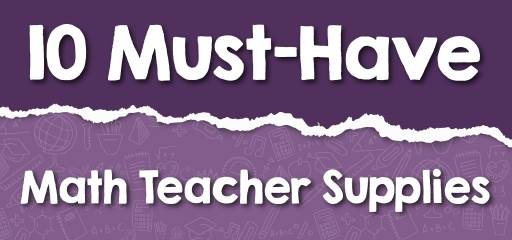# 10 Must-Have Math Teacher SuppliesAs you may know, although mathematics can be a very engaging lesson, to achieve such a feeling, students must first be able to communicate with mathematics. It is up to the teacher to make the right connection between the students and the math lesson in the classroom. To create such a pleasant connection, teachers can use supplies that make it easier to understand mathematical concepts. Therefore, in this article, we have reviewed 10 must-have math teacher supplies.

## 1-Calculators

It may happen to you that when teaching, you come across topics that need to be well understood by students, regardless of computational skills. In such cases, calculators can be used to speed up the teaching math process. In fact, calculators can save teachers time to spend on more important content by performing complex calculations unrelated to the current educational topic.

## 2-Geometric Shapes

It will be easier to teach the concepts of geometric shapes using geometric solids. Using geometric tools, students can better understand and visualize geometric features. Each geometric shape has its own sides and formulas that will be easier to memorize by using the corresponding shape solids. In this case, students can clearly see the abstract concepts that you present.

## 3-Rulers

Rulers have many uses: measuring or helping to draw a straight line. No one can ignore the role of rulers in understanding and learning mathematical concepts. Rulers are also useful for teaching students the relationship between metric and customary units. Although rulers may seem like simple tools, their absence in math classrooms can make a big difference. 44

## 4-Number Line Sets

Using the number line sets will allow you to teach integer topics to your students in a more engaging way. It can also be very helpful in teaching absolute value as well as comparing integers. The number line is one of the things that is very effective in visual teaching of those mathematics concepts that may seem boring.

## 5-Teaching Dice

Teaching Dice are a type of dice that have different numbers on each side and can be used to teach students the greatest common factor and the least common multiple. These dice are also a good tool for teaching probabilities and statistics. These tools are not only suitable for teaching math to students in the classroom but are also a good option for practicing math at home.

## 6-Magnetic Fraction Tiles

Learning the concepts of fractions and decimals can be very easy using Magnetic Fraction Tiles. Using these magnetic tiles provides a fun, practical way to teach fractions. In addition, they are great for a whiteboard demonstration and are used to present fraction lessons engagingly. Therefore, when introducing any fraction or decimal operation, these magnetic tiles can be used to make learning easy and fun for students.

## 7-Integer Counters

Integer Counters are a good visual tool for introducing addition and subtraction with integers. You can also use them instead of coins in probabilities. These counters are available in a variety of foams and plastics and can be used to improve students’ counting skills. So, if you do not know how to effectively teach your students the integers operation, you can use these Counters to better understand the subject.

## 8-Math Playing Cards

Math playing cards are used to teach basic math concepts and can be used to teach addition, subtraction, multiplication, and probability to beginners. Using these cards is a simple and effective way to learn basic math concepts. In fact, these cards use competition and entertainment to help your students learn math. In addition, these cards help increase students’ speed in solving math problems.

## 9-Algebra Foam Tiles

Using algebra foam tiles, you can have fun explaining to students how to simplify expressions. It is also possible to combine like terms with the help of algebra tiles. With the help of these foam tiles, students can make polynomial geometric models and thus better understand algebraic concepts.

## 10-Balance

Balance can be used to explain the properties of equality to students. When teaching equation solving, balance is used to show the equality of expressions on each side. Thus, the use of balance is a practical way to introduce mass and measurement in the classroom.

Finally, be creative and make math easy! The tools in this article are just a few of the dozens of items you can use to better teach math to your students. The world of math is vast and with a little creativity, you can make learning math more enjoyable.

### What people say about "10 Must-Have Math Teacher Supplies - Effortless Math: We Help Students Learn to LOVE Mathematics"?

No one replied yet.

X
30% OFF

Limited time only!

Save Over 30%

SAVE \$5

It was \$16.99 now it is \$11.99